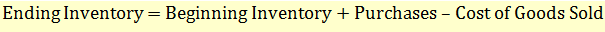# Inventory Valuation Methods

Recall the inventory equation:If the cost of inventory remained constant over time, then the valuation of inventories would be very simple. We will simply multiply the number of units sold with the cost of each unit to calculate the cost of goods sold (COGS). Similarly the value of ending inventory would be the number of units in inventory multiplied by the cost of each unit. However, in reality, the cost of inventory changes over time, and we must choose an inventory valuation method to allocate the various costs to COGS (income statement) and ending inventory (balance sheet). These methods are called cost flow methods.

The four inventory valuation methods are:

• Specific identification
• First in, first out (FIFO)
• Last in, first out (LIFO)
• Weighted Average Cost

Under US GAAP, all these methods are permitted. Under IFRS, LIFO method is not allowed.

Under these methods:

• The ending inventory in terms of units will be the same
• The COGS and cost of ending inventory will be different
• Purchase cost will be same
• If the costs are rising, LIFO results in lowest net income

We will take an example to explain inventory valuation under all four methods. Let’s say SuperMart reports the following transactions for June.